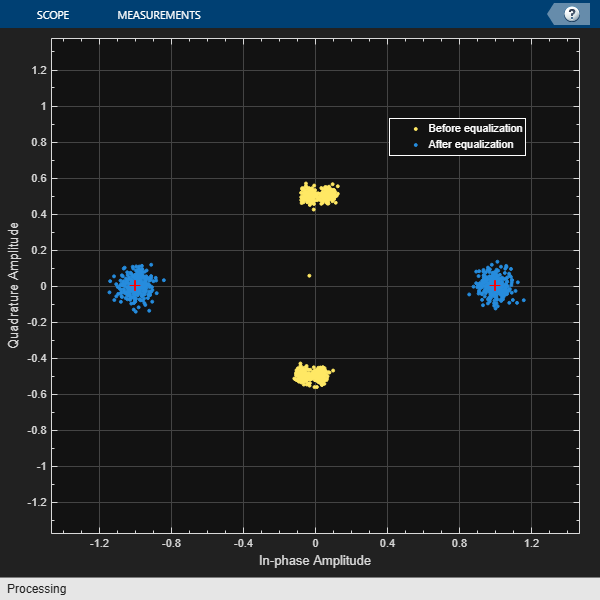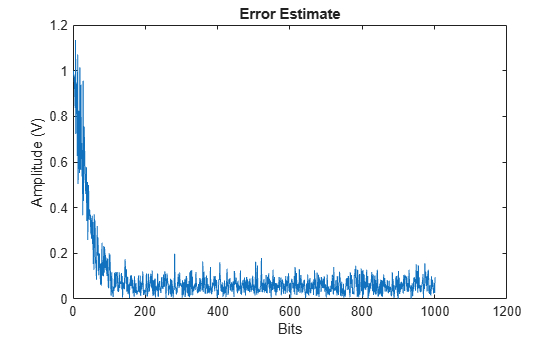Documentation

## Equalize BSPK Signal

Equalize a BPSK signal using a linear equalizer with an least mean square (LMS) algorithm.

Generate random binary data and apply BPSK modulation.

```data = randi([0 1],1000,1); modData = pskmod(data,2);```

Apply two-tap static fading to the modulated signal.

`rxSig = conv(modData,[0.5 0.05]);`

Create an LMS adaptive algorithm object with a step size of 0.06.

`alg = lms(0.06);`

Create a linear equalizer object having 8 taps using the previously created algorithm object. Set the reference tap index to 4.

```eqlms = lineareq(8,alg); eqlms.RefTap = 4;```

Equalize the received signal, `rxSig`, while using the first 200 data bits as a training sequence.

```trSeq = data(1:200); [eqSig,~,e] = equalize(eqlms,rxSig,trSeq);```

Filter and plot the power of the received (nonequalized) signal. The magnitude of the signal has been attenuated by the channel.

```rxSigPwr = filter(0.1*ones(10,1),1,abs(rxSig)).^2; plot(rxSigPwr) title('Received Signal') xlabel('Bits') ylabel('Power (W)')```Plot the equalized signal. The signal reaches the intended power level of 1 W.

```eqSigPwr = filter(0.1*ones(10,1),1,abs(eqSig)).^2; plot(eqSigPwr) title('Equalized Signal') xlabel('Bits') ylabel('Power (W)')```Plot the magnitude of the error estimate, `e`. The error decreases until it is nearly zero after 400 bits.

```plot(abs(e)) title('Error Estimate') xlabel('Bits') ylabel('Amplitude (V)')```